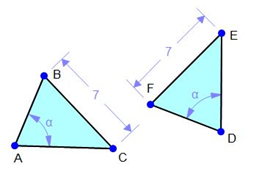##### KAPEC GEOTASK?
• The learning process is entertaining and exciting
• The solved tasks turn into coordinates
• The chance to follow your progress
• Along with your schoolmates and friends, go on an adventure, searching for hideouts
• E-mail:

# 2379.2. Equal triangles, equal triangle appropriate elements, similar properties and signs#### Tick the correct answer!

Is it possible to prove that the presented triangles are equal if using triangle equality signs and already known coherences in triangle (sum of all triangle inner angles is 180 degrees)?

#### Next task: 2378. Equal triangles, equal triangle appropriate elements, similar properties and signs

Grade:
7
Subject
Geometry
Theme
Equal triangles.
Subtheme
Equal triangles, appropriate elementrs, similar properties and signs.

#### Solve tasks to find hidden Geo point coordinates